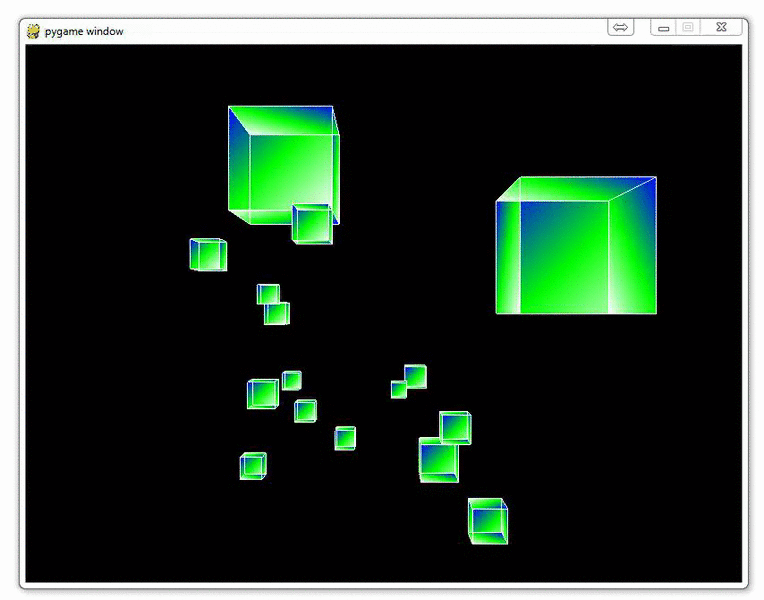## Infinite flying cubes

Let's all be honest with each other. We all aspire to create screen-savers, and boy do I have a really neat screensaver idea. Infinite flying cubes, like the infinite "stars" screen-saver! We can definitely sell this idea and make the big bucks!

We've seen that we have a bit of an issue with processing a lot of cubes. Either the game is really short with many cubes, or the game is longer but with very few cubes at a time. Depending on your computer's processing ability, you may get away with more or less than I have in the videos, but this is relatively problematic. Our game is extremely fixed in length. This is okay, but, for a game like the one we have, we can actually do the processing as the game proceeds, generating the coordinates for cubes as we go. There's no real reason to retain cubes that we have passed in memory, nor is there to have cubes at an immense distance away from us in memory.

So what we're going to do here is randomly generate new cubes as needed, and get rid of cubes that we have passed along the way.

I will most most of the major changes here, then post the full code at the bottom, since it can be confusing to edit your own code from these chunks and I might miss one of the changes.

First, we need to add a new parameter to set_vertices:

```def set_vertices(max_distance,min_distance = -20):
x_value_change = random.randrange(-15,15)
y_value_change = random.randrange(-15,15)
...rest of the code...
```

We define a new minimum distance.

If you're following from the previous video with the ground, comment out the ground function in the main loop.

Next, after the for loop that creates the cubes:

```        for each_cube in cube_dict:
Cubes(cube_dict[each_cube])
```

```        for each_cube in cube_dict:
if camera_z <= cube_dict[each_cube]:
print("passed a cube")
#delete_list.append(each_cube)
new_max = int(-1*(camera_z-max_distance))

cube_dict[each_cube] = set_vertices(new_max,int(camera_z))

```

What we're doing here is first removing any cube from the dictionary that we've passed. From there, we're adding a new cube to take its place. What this allows us to do is only have, in our case, 60 cubes at a time, yet continue to go on and on using a sort of recycling method.

The full code for this is:

```import pygame
from pygame.locals import *

from OpenGL.GL import *
from OpenGL.GLU import *

import random

vertices = (
(1, -1, -1),
(1, 1, -1),
(-1, 1, -1),
(-1, -1, -1),
(1, -1, 1),
(1, 1, 1),
(-1, -1, 1),
(-1, 1, 1)
)

edges = (
(0,1),
(0,3),
(0,4),
(2,1),
(2,3),
(2,7),
(6,3),
(6,4),
(6,7),
(5,1),
(5,4),
(5,7)
)

surfaces = (
(0,1,2,3),
(3,2,7,6),
(6,7,5,4),
(4,5,1,0),
(1,5,7,2),
(4,0,3,6)
)

colors = (
(1,0,0),
(0,1,0),
(0,0,1),
(0,1,0),
(1,1,1),
(0,1,1),
(1,0,0),
(0,1,0),
(0,0,1),
(1,0,0),
(1,1,1),
(0,1,1),
)

##ground_vertices = (
##    (-10, -1.1, 20),
##    (10, -1.1, 20),
##    (-10, -1.1, -300),
##    (10, -1.1, -300),
##    )
##
##
##def ground():
##    for vertex in ground_vertices:
##        glColor3fv((0,0.5,0.5))
##        glVertex3fv(vertex)
##
##    glEnd()

def set_vertices(max_distance, min_distance = -20):
x_value_change = random.randrange(-10,10)
y_value_change = random.randrange(-10,10)
z_value_change = random.randrange(-1*max_distance,min_distance)

new_vertices = []

for vert in vertices:
new_vert = []

new_x = vert + x_value_change
new_y = vert + y_value_change
new_z = vert + z_value_change

new_vert.append(new_x)
new_vert.append(new_y)
new_vert.append(new_z)

new_vertices.append(new_vert)

return new_vertices

def Cube(vertices):

for surface in surfaces:
x = 0

for vertex in surface:
x+=1
glColor3fv(colors[x])
glVertex3fv(vertices[vertex])

glEnd()

glBegin(GL_LINES)
for edge in edges:
for vertex in edge:
glVertex3fv(vertices[vertex])
glEnd()

def main():
pygame.init()
display = (800,600)
pygame.display.set_mode(display, DOUBLEBUF|OPENGL)

max_distance = 100

gluPerspective(45, (display/display), 0.1, max_distance)

glTranslatef(random.randrange(-5,5),random.randrange(-5,5), -40)

#object_passed = False

x_move = 0
y_move = 0

cube_dict = {}

for x in range(20):
cube_dict[x] =set_vertices(max_distance)

#glRotatef(25, 2, 1, 0)

while True:
for event in pygame.event.get():
if event.type == pygame.QUIT:
pygame.quit()
quit()

if event.type == pygame.KEYDOWN:
if event.key == pygame.K_LEFT:
x_move = 0.3
if event.key == pygame.K_RIGHT:
x_move = -0.3

if event.key == pygame.K_UP:
y_move = -0.3
if event.key == pygame.K_DOWN:
y_move = 0.3

if event.type == pygame.KEYUP:
if event.key == pygame.K_LEFT or event.key == pygame.K_RIGHT:
x_move = 0

if event.key == pygame.K_UP or event.key == pygame.K_DOWN:
y_move = 0

##            if event.type == pygame.MOUSEBUTTONDOWN:
##                if event.button == 4:
##                    glTranslatef(0,0,1.0)
##
##                if event.button == 5:
##                    glTranslatef(0,0,-1.0)

x = glGetDoublev(GL_MODELVIEW_MATRIX)

camera_x = x
camera_y = x
camera_z = x

glClear(GL_COLOR_BUFFER_BIT|GL_DEPTH_BUFFER_BIT)

glTranslatef(x_move,y_move,.50)

#ground()

for each_cube in cube_dict:
Cube(cube_dict[each_cube])

for each_cube in cube_dict:
if camera_z <= cube_dict[each_cube]:
print("passed a cube")
#delete_list.append(each_cube)
new_max = int(-1*(camera_z-max_distance))

cube_dict[each_cube] = set_vertices(new_max,int(camera_z))

pygame.display.flip()
pygame.time.wait(10)

main()
pygame.quit()
quit()
```

The next tutorial:• OpenGL with PyOpenGL introduction and creation of Rotating Cube

• Coloring Surfaces as well as understand some of the basic OpenGL code

• Understanding navigation within the 3D environment via OpenGL

• Moving the player automatically towards the cube

• Random Cube Position

• Adding Many Cubes to our Game

• Adding a ground in OpenGL

• Infinite flying cubes
• Optimizing the processing for infinite cubes